# What is a Keras Model

This Python tutorial is a part of our series of Python packages related tutorials.

Keras is a neural network Application Programming Interface (API) for Python that is tightly integrated with TensorFlow, which is used to build machine learning models. Keras’ models offer a simple, user-friendly way to define a neural network, which will then be built for you by TensorFlow.

#### What’s the Difference Between Tensorflow and Keras?

TensorFlow is an open-source set of libraries for creating and working with neural networks, such as those used in Machine Learning (ML) and Deep Learning projects.

Keras, on the other hand, is a high-level API that runs on top of TensorFlow. Keras simplifies the implementation of complex neural networks with its easy to use framework.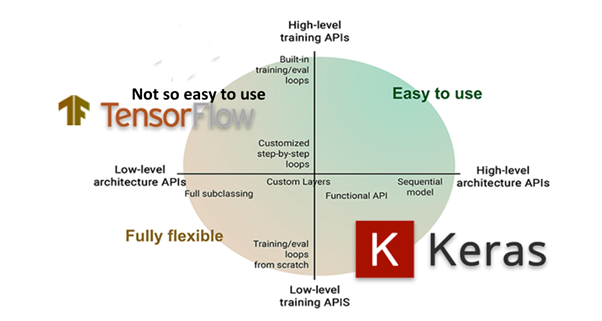Figure 1: TensorFlow vs Keras

### When to Use Keras vs TensorFlow

TensorFlow provides a comprehensive machine learning platform that offers both high level and low level capabilities for building and deploying machine learning models. However, it does have a steep learning curve. It’s best used when you have a need for:

• Deep learning research
• Complex neural networks
• Working with large datasets
• High performance models

Keras, on the other hand, is perfect for those that do not have a strong background in Deep Learning, but still want to work with neural networks. Using Keras, you can build a neural network model quickly and easily using minimal code, allowing for rapid prototyping. For example:

```# Import the Keras libraries required in this example:
from keras.models import Sequential
from keras.layers import Dense, Activation

# Create a Sequential model:
model = Sequential()

# Add layers with the add() method:
model.add(Dense(32, input_dim=784))
model.add(Activation('relu'))```

Keras is less error prone than TensorFlow, and models are more likely to be accurate with Keras than with TensorFlow. This is because Keras operates within the limitations of its framework, which include:

• Computation speed: Keras sacrifices speed for user-friendliness.
• Low-level Errors: sometimes you’ll get TensorFlow backend error messages that Keras was not designed to handle.
• Algorithm Support – Keras is not well suited for working with certain basic machine learning algorithms and models like clustering and Principal Component Analysis (PCM).
• Dynamic Charts – Keras has no support for dynamic chart creation.

## Keras Model Overview

Models are the core entity you’ll be working with when using Keras. The models are used to define TensorFlow neural networks by specifying the attributes, functions, and layers you want.

Keras offers a number of APIs you can use to define your neural network, including:

• Sequential API, which lets you create a model layer by layer for most problems. It’s straightforward (just a simple list of layers), but it’s limited to single-input, single-output stacks of layers.
• Functional API, which is a full-featured API that supports arbitrary model architectures. It’s more flexible and complex than the sequential API.
• Model Subclassing, which lets you implement everything from scratch. Suitable for research and highly complex use cases, but rarely used in practice.

### How to Define a Neural Network with Keras’ Sequential API

The Sequential API is a framework for creating models based on instances of the sequential() class. The model has one input variable, a hidden layer with two neurons, and an output layer with one binary output. Additional layers can be created and added to the model.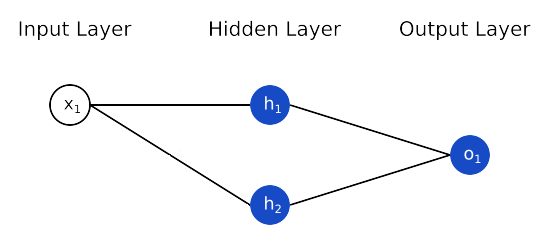Figure 2: A Simple Model

```# Define the model:
from keras.models import Sequential
from keras.layers import Dense
model = Sequential()
model.add(Dense(2, input_dim=1, activation='relu'))
model.add(Dense(1, activation='sigmoid'))```

The model includes the following information:

• Layers and their order in the model.
• Output shape (number of elements in each dimension of output data)  of each layer.
• Number of parameters (weights) in each layer.
• Total number of parameters in the model.

The summary() function is used to generate and print the summary in the Python console:

```# Print a summary of the created model:
from keras.models import Sequential
from keras.layers import Dense
model = Sequential()
model.add(Dense(2, input_dim=1, activation='relu'))
model.add(Dense(1, activation='sigmoid'))
print(model.summary())```

Output: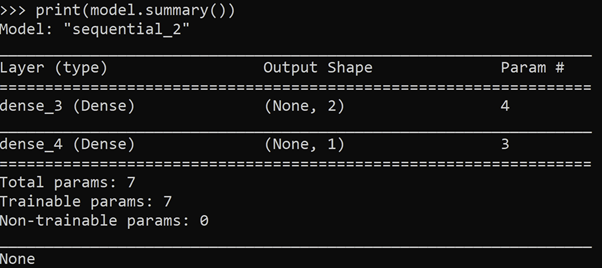```# Add and define multiple layers and pass them
# to the Sequential model as an array:
from keras.models import Sequential
from keras.layers import Dense
model = Sequential([Dense(2, input_dim=1), Dense(1)])```
```# Define a single layer and add it to the Sequential model:
from keras.models import Sequential
from keras.layers import Dense
model = Sequential()
model.add(Dense(2, input_dim=1))
model.add(Dense(1))
```

### How to Define a Neural Network with Keras’ Functional API

The Keras functional API lets you:

• Define multiple input or output models
• Define models that share layers
• Create an acyclic network graph

Functional API models are defined by creating instances of layers, and connecting them directly to each other in pairs. A model is then defined that specifies the layers to act as the input and output to the model.

#### Create an Input Layer

In the Functional API model, unlike the Sequential API model, you must first create and define a standalone input layer that specifies the shape of input data.

The input layer takes a shape argument that is a tuple representing the dimension of the input data. When input data is one-dimensional, the shape must explicitly leave room for the shape of a mini-batch size used when splitting the data when training the network. Therefore, the shape tuple is always defined with a hanging last dimension, eg. (2,).

```# Define the input layer:
from keras.layers import Input
visible = Input(shape=(2,))```

Layers in the model are connected pairwise by specifying where the input comes from when defining each new layer. A bracket notation is used, specifying the input layer.

```# Connect the layers, then create a hidden layer as a Dense
# that receives input only from the input layer:
from keras.layers import Dense
visible = Input(shape=(2,))
hidden = Dense(2)(visible)```

The Functional API model gets its flexibility by connecting layers piece by piece in this manner.

#### Create a Model

The Functional API provides a model() class for creating a model from your layers. It requires that you specify input and output layers.

```# Define a Functional API model:
from keras.models import Model
from keras.layers import Input
from keras.layers import Dense
visible = Input(shape=(2,))
hidden = Dense(2)(visible)
model = Model(inputs=visible, outputs=hidden)```

## How to Use Keras Models to Make Predictions

After a model is defined with either the Sequential or Functional API, various functions need to be created in preparation for training and fitting a model, before we can use it to make a prediction:

In this example, a Keras Sequential model is implemented to fit and predict regression data:

```# PREPARE THE DATA

# Import libraries required in this example:
import random
import numpy as np
import matplotlib.pyplot as plt
from keras.models import Sequential
from keras.layers import Dense
from keras.wrappers.scikit_learn import KerasRegressor
from sklearn.metrics import mean_squared_error

# Generate a sample dataset from random data:
random.seed(123)
def CreateDataset(N):
a,b,c,y = [],[],[],[]
for i in range(N):
aa = i/10+random.uniform(-4,3)
bb = i/30+random.uniform(-4,4)
cc = i/40+random.uniform(-3,3)-5
yy = (aa+bb+cc/2)/3
a.append([aa])
b.append([bb])
c.append([cc])
y.append([yy])
return np.hstack([a,b,c]), np.array(y)

N = 150
x,y = CreateDataset(N)
x_ax = range(N)
plt.plot(x_ax, x, 'o', label="original value", markersize=3)
plt.plot(x_ax, y, lw=1.5, color="red", label="y")
plt.legend(['original value'])
plt.show()
```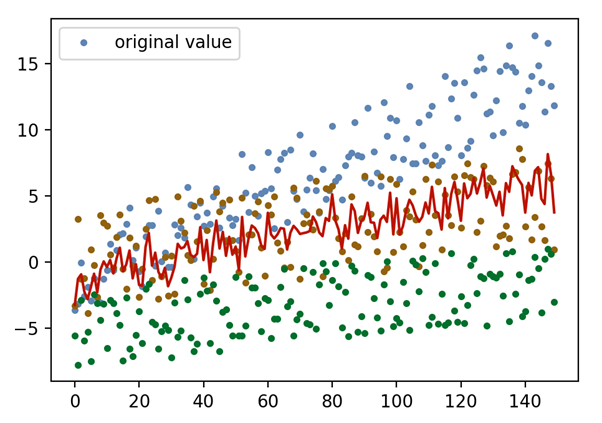Figure 3: Sample dataset

```# Define and build a Sequential model, and print a summary:
def BuildModel():
model = Sequential()
model.add(Dense(128, input_dim=3,activation='relu'))
model.add(Dense(32, activation='relu'))
model.add(Dense(8,activation='relu'))
model.add(Dense(1,activation='linear'))
model.compile(loss="mean_squared_error", optimizer="adam")
return model

BuildModel().summary()```
```# Fit the Sequential model with Scikit-learn Regressor API for Keras:
regressor = KerasRegressor(build_fn=BuildModel,nb_epoch=100,batch_size=3)
regressor.fit(x,y)

y_pred = regressor.predict(x)
mse_krr = mean_squared_error(y, y_pred)
print(mse_krr)

plt.plot(y, label="y-original")
plt.plot(y_pred, label="y-predicted")
plt.legend()
plt.show()```

```# Fit the model without the KerasRegressor wrapper:
model = BuildModel()
model.fit(x, y, nb_epoch=100, verbose=False, shuffle=False)
y_krm = model.predict(x)
mse_krm=mean_squared_error(y, y_krm)
print(mse_krm)

plt.plot(y)
plt.plot(y_krm, label="y-predicted")
plt.legend()
plt.show()```

### Machine Learning Concepts and Terminology

• Accuracy. Calculates the percentage of predicted values (yPred) that match actual values (yTrue).
• Batch. A set of N samples. Each sample in a batch is processed independently, in parallel with the other samples. Commonly referred to as a mini-batch.
• Batch Size. Number of samples processed through to the network at one time.
• Convolutional Neural Network (CNN, or ConvNet). Class of deep neural networks, commonly applied to analysis of visual imagery. Inspired by biological processes.
• Epoch. One single pass over the entire training set to the network. An arbitrary cutoff in training, defined as ‘one pass over the entire dataset’.
• GPU. Graphics Processing Unit. A TensorFlow processor platform that shows better flexibility and programmability for irregular computations, such as small batches. NVidia CUDA card requirement.
• Gradient. Slope of a function. Gradient measures the change in all weights with regard to the change in error.
• Layer. Instances of the layer() class are the basic building blocks in Keras neural networks. Consists of a tensor-in tensor-out computation function (the layer’s call method) and some state, held in TensorFlow variables.
• Loss (L). Measure of how far a model’s predictions are from its label. Metric that represents how good/bad a model is. Objective is to find a set of weights and biases that minimize loss. To determine loss, a model defines a loss function. Linear regression models typically use mean squared error while logistic regression models use Log Loss, for loss function.

Loss functions are available in the losses library. One of two required arguments for compiling a Keras model. To import the losses library, enter:
`from keras import losses`

Partial list of available loss functions:

```mean_squared_error
mean_absolute _error
hinge
mean_absolute_percentage _error
mean_squared_logarithmic_error
Poisson
binary_crossentropy
categorical_crossentropy```
• Metrics. Specify the evaluation criteria for the model. To import the metrics library, enter:
`    from keras import metrics`

Available metrics:

```accuracy
binary_accuracy
categorical_accuracy
cosine_proximity
clone_metric```
• Neural Network. System of algorithmic methods for recognizing predictive relationships in data, in a process that mimics the neural patterns of the human brain.
• Neuron. Basic building block of an artificial neural network. Biological analogy of neurons in the human brain, in which neurons (cells that act as fundamental units in a neural nervous system) send/receive input and output.
• One-Hot Encoding. Process in which categorical variables are converted into a suitable format for ML prediction.
• OptimizerOptimizer/loss functions are used to minimize loss. Optimizers are used to adjust input weights, by comparing prediction and the loss function. To import the optimizers library, enter:
`from keras import optimizers`

Available optimizers:

```SGD (Stochastic Gradient Descent)
RMSprop
Adagrad
Adam
Adamax
Nadam```

To specify the learning rate for the Adam optimizer, enter:

`keras.optimizers.Adam(learning_rate=0.001)`

To compile a Keras model:

`model.compile(loss="mean_squared_error", optimizer="adam")`
• Rank. Number of dimensions in a tensor. For instance, a scalar has rank 0, a vector has rank 1, and a matrix has rank 2.
• Sample. One element of a dataset. Eg. One image in a convolutional neural network.
• Shape. List or tuple of numbers containing the size of each dimension of a tensor object. First layer in a Sequential model needs to receive information about its ‘input shape’. input_shape argument creates a tuple containing one element. Tensor shape notation:
• N-dimensional tensor: (D0, D1, …, Dn-1)
• Matrix tensor of size W x H: (W, H)
• Vector tensor of size W: (W,)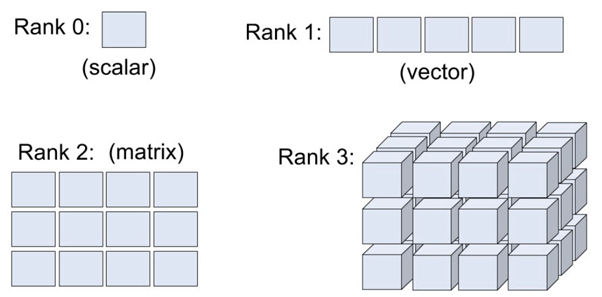Figure 4: Tensor Shape

• Tensor. Generalization of vectors and matrices that are n-dimensional and contain the same type of data, eg. int32 or bool, etc. A Keras tensor is a TensorFlow symbolic tensor object. Tensors are the primary data structure in TensorFlow and in neural networks. (Compare ‘generalization’ of vectors in Keras/TensorFlow with ‘summation’ of vectors in tensor calculus.)
In terms of notation, whether its TensorFlow or tensor calculus, tensors occur when more than two indices are required to express elements in a scalar, vector, or matrix.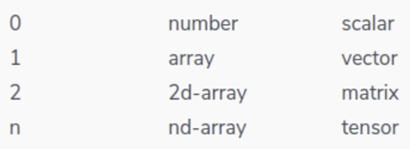Figure 5: Indices – Scalar, Vector, Matrix, Tensor objects

• Tensor Processing Unit (TPU).  Programmable AI accelerator designed to provide high throughput of low-precision arithmetic. A TensorFlow processor platform that is highly-optimised for large batches and CNNs, with high training throughput. A TPU platform typically consists of multiple TPU devices connected to each other over a dedicated high-speed network connection. Available on Google’s Colaboratory (Colab) platform.
• Variable. A tensorFlow variable represents a tensor whose values can be changed by running ops on it. Specific ops allow you to read and modify the values of a specified tensor.
• Weights and Biases (W & B).
• Weights. Input parameter that influences output in a Keras model.
• Biases are an extra threshold value added to the output.
• In general statistics, weights express an increase/decrease in the importance or magnitude of an item. While biases are arbitrary values denoting incorrect or undesirable weights.

The following tutorials will provide you with step-by-step instructions on how to work with machine learning Python packages:

### Get a version of Python, pre-compiled with Keras and other popular ML Packages

ActivePython is the trusted Python distribution for Windows, Linux and Mac, pre-bundled with top Python packages for machine learning – free for development use.

### Some Popular ML Packages You Get Pre-compiled – With ActivePython

Machine Learning:

• TensorFlow (deep learning with neural networks)*
• scikit-learn (machine learning algorithms)
• keras (high-level neural networks API)

Data Science:

• pandas (data analysis)
• NumPy (multidimensional arrays)
• SciPy (algorithms to use with numpy)
• HDF5 (store & manipulate data)
• matplotlib (data visualization)

Get ActivePython for Machine Learning for Windows, macOS or Linux here.

#### Why use ActivePython instead of open source Python?

While the open source distribution of Python may be satisfactory for an individual, it doesn’t always meet the support, security, or platform requirements of large organizations.

This is why organizations choose ActivePython for their data science, big data processing and statistical analysis needs.

Pre-bundled with the most important packages Data Scientists need, ActivePython is pre-compiled so you and your team don’t have to waste time configuring the open source distribution. You can focus on what’s important–spending more time building algorithms and predictive models against your big data sources, and less time on system configuration.

ActivePython is 100% compatible with the open source Python distribution and provides the security and commercial support that your organization requires.

With ActivePython you can explore and manipulate data, run statistical analysis, and deliver visualizations to share insights with your business users and executives sooner–no matter where your data lives.

Download ActivePython Community Edition to get started or contact us to learn more about using ActivePython in your organization.

#### Related Python Use Cases:

How to monitor social distancing using Python and object detectionUse ActivePython and accelerate your Python projects.

• The #1 Python solution used by innovative enterprise teams
• Comes pre-bundled with top Python packages
• Spend less time resolving dependencies and more time on quality coding

Remi M